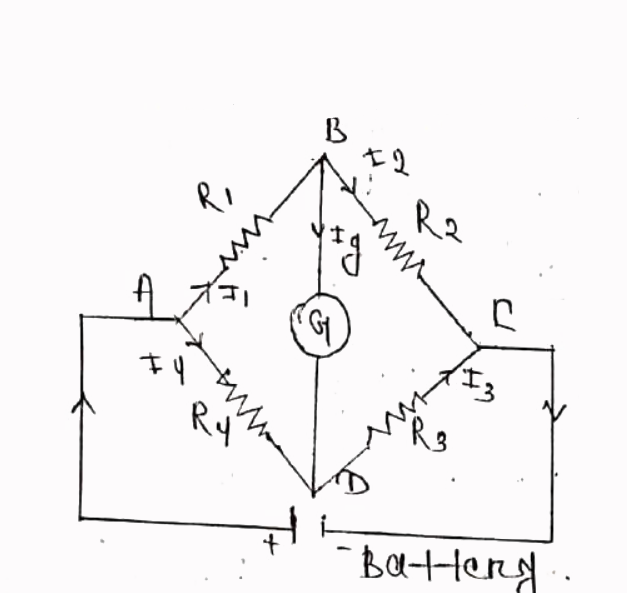### WheatStone Bridge Method

The Wheatstone Bridge is one of the most common and simplest bridge networks / circuits, which can be used to measure resistance very precisely. But often the Wheatstone Bridge is used with Transducers to measure physical quantities like Temperature, Pressure, Strain etc.

Wheatstone Bridge is used in applications where small changes in resistance are to be measured in sensors. This is used to convert a change in resistance to a change in voltage of a transducer. The combination of this bridge with the operational amplifier is used extensively in industries for various transducers and sensors.For example, the resistance of a Thermistor changes when it is subjected to change in temperature. Likewise, a strain gauge, when subjected to pressure, force or displacement, its resistance changes. Depending on the type of application, the Wheatstone Bridge can be operated either in a Balanced condition or an Unbalanced condition.A Wheatstone bridge consists of four resistors (R1, R2, R3 and R4) that are connected in the shape of a diamond with the DC supply source connected across the top and bottom points (C and D in the circuit) of the diamond and the output is taken across the other two ends (A and B in the circuit).This bridge is used to find the unknown resistance very precisely by comparing it with a known value of resistances. In this bridge, a Null or Balanced condition is used to find the unknown resistance.For this bridge to be in a Balanced Condition, the output voltage at points A and B must be equal to 0. From the above circuit:

The Bridge is in Balanced Condition if:

VOUT = 0 V

Now, for Balanced Condition, the voltage across the resistors R1 and R2 is equal. If V1 is the voltage across R1 and V2 is the voltage across R2, then:

V1 = V2

Similarly, the voltage across resistors R3 (let us call it V3) and R4 ( let us call it V4) are also equal. So,

V3 = V4

The ratios of the voltage can be written as:

V1 / V3 = V2 / V

From Ohm’s law, we get:

I1 R1 / I3 R3 = I2 R2 / I4 R4

Since I1 = I3 and I2 = I4,

we get:

R1 / R3 = R2 / R4

From the above equation, if we know the values of three resistors, we can easily calculate the resistance of the fourth resistor.

Find Unknown Resistance using Balanced Wheatstone Bridge
In the above circuit, let us assume that R1 is an unknown resistor. So, let us call it RX. The resistors R2 and R4 have a fixed value. Which means, the ratio R2 / R4 is also fixed. Now, from the above calculation, to create a balanced condition, the ratio of resistors must be equal i.e.,
RX / R3 = R2 / R

Since the ratio R2 / R4 is fixed, we can easily adjust the other known resistor (R3) to achieve the above condition. Hence, it is important that R3 is a variable resistor, which we call RV.

By placing the Galvanometer between the points A and B, we can detect the Balanced Condition.With RX placed in the circuit, adjust the RV until the Galvanometer points to 0. At this point, note down the value of RV. By using the following formula, we can calculate the unknown resistor RX.

RX = RV (R/ R4)